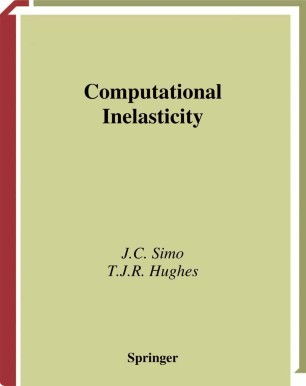# Computational InelasticityBook

Part of the Interdisciplinary Applied Mathematics book series (IAM, volume 7)

1. Front Matter
Pages i-xiv
2. Pages 336-373
3. Back Matter
Pages 375-392

### Introduction

This book describes the theoretical foundations of inelasticity, its numerical formulation and implementation. The subject matter described herein constitutes a representative sample of state-of-the- art methodology currently used in inelastic calculations. Among the numerous topics covered are small deformation plasticity and viscoplasticity, convex optimization theory, integration algorithms for the constitutive equation of plasticity and viscoplasticity, the variational setting of boundary value problems and discretization by finite element methods. Also addressed are the generalization of the theory to non-smooth yield surface, mathematical numerical analysis issues of general return mapping algorithms, the generalization to finite-strain inelasticity theory, objective integration algorithms for rate constitutive equations, the theory of hyperelastic-based plasticity models and small and large deformation viscoelasticity. Computational Inelasticity will be of great interest to researchers and graduate students in various branches of engineering, especially civil, aeronautical and mechanical, and applied mathematics.

### Keywords

Applied Mathematics Calculation Finite Mathematica algorithms calculus equation finite element method mathematics numerical analysis optimization

#### Authors and affiliations

1. 1.Stanford UniversityStanfordUSA
2. 2.Mechanics and Computation Durand BuildingStanford UniversityStanfordUSA

### Bibliographic information

• Book Title Computational Inelasticity
• Authors J.C. Simo
T.J.R. Hughes
• Series Title Interdisciplinary Applied Mathematics
• DOI https://doi.org/10.1007/b98904
• Copyright Information Springer-Verlag New York, Inc. 1998
• Publisher Name Springer, New York, NY
• eBook Packages
• Hardcover ISBN 978-0-387-97520-7
• Softcover ISBN 978-1-4757-7169-5
• eBook ISBN 978-0-387-22763-4
• Series ISSN 0939-6047
• Edition Number 1
• Number of Pages XIV, 392
• Number of Illustrations 0 b/w illustrations, 0 illustrations in colour
• Topics
• Buy this book on publisher's site

## Reviews

"This book is well written, is easy to follow, and contains much useful material for researchers and graduate students in various branches of engineering and applied mathematics." Newsletter of the EMS, Issue 42, December 2001